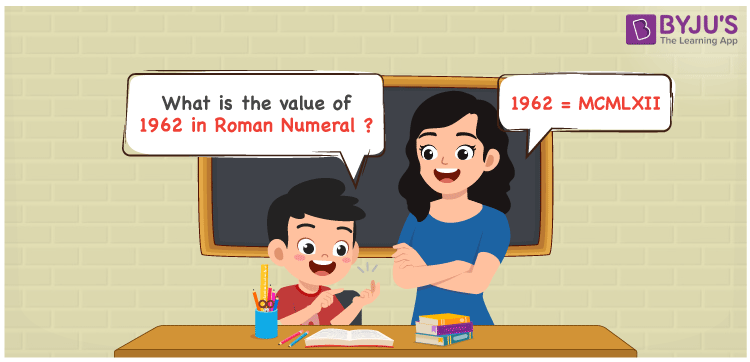# 1962 in Roman Numerals

1962 in Roman Numerals is MCMLXII. The system of writing numbers with the help of the Roman alphabet is known as Roman Numerals. Students can access Roman Numerals 1 to 100 in PDF format to solve any type of problem with ease irrespective of their intelligence quotient and score good marks in exams. In this article, we will learn how to express 1962 in Roman Numerals in a precise manner.

 Number Roman Numeral 1962 MCMLXII

## How to Write 1962 in Roman Numerals?The following method can be used to express 1962 in Roman Numerals

• Break the number 1962 into the simplest form
• 1962 = 1000 + (1000 – 100) + 50 + 10 + 1 + 1
• Write their respective Roman letter and add/subtract them
• 1962 = M + (M – C) + L + X + I + I
• Thus the value of 1962 in Roman Numerals is MCMLXII

## Video Lesson on Roman Numerals## Related Articles

Roman Numerals

Roman Numerals 1 to 50

Roman Numerals 1 to 1000

Roman Numerals Conversion

L Roman Numeral

Roman Numeral XXXIX

## Frequently Asked Questions on 1962 in Roman Numerals

Q1

### What is 1962 in Roman Numerals?

1962 in Roman Numerals is MCMLXII.
Q2

### What is the value of 1900 + 62? Express the answer in Roman Numerals.

1900 + 62 = 1962. The number 1962 in Roman Numerals is MCMLXII. Hence the value of 1900 + 62 in Roman Numerals is MCMLXII.
Q3

### Find the difference between 2050 and 1962 in Roman Numerals.

2050 – 1962 = 88. The number 88 in Roman Numerals is LXXXVIII. Therefore the difference between 2050 and 1962 in Roman Numerals is LXXXVIII.